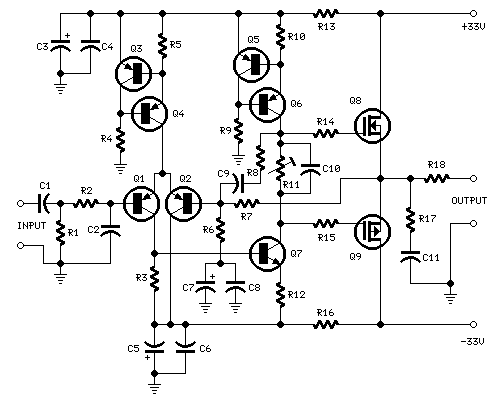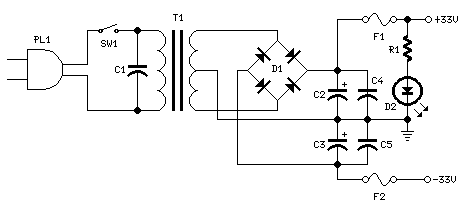# How to build 25W Mosfet audio amplifier

High Quality simple unit
No need for a preamplifier

circuit diagramParts:
R1,R4 = 47K 1/4W Resistors
R2 = 4K7 1/4W Resistors
R3 = 1K5 1/4W Resistors
R5 = 390R 1/4W Resistors
R6 = 470R 1/4W Resistors
R7 = 33K 1/4W Resistors
R8 = 150K 1/4W Resistors
R9 = 15K 1/4W Resistors
R10 = 27R 1/4W Resistors
R11 = 500R 1/2W Trimmer Cermet
R12,R13,R16 = 10R 1/4W Resistors
R14,R15 = 220R 1/4W Resistors
R17 = 8R2 2W Resistor
R18 = R22 4W Resistor (wirewound)
C1 = 470nF 63V Polyester Capacitor
C2 = 330pF 63V Polystyrene Capacitor
C3,C5 = 470uF 63V Electrolytic Capacitors
C4,C6,C8,C11 = 100nF 63V Polyester Capacitors
C7 = 100uF 25V Electrolytic Capacitor
C9 = 10pF 63V Polystyrene Capacitor
C10 = 1uF 63V Polyester Capacitor
Q1-Q5 = BC560C 45V100mA Low noise High gain PNP Transistors
Q6 = BD140 80V 1.5A PNP Transistor
Q7 = BD139 80V 1.5A NPN Transistor
Q8 = IRF532 100V 12A N-Channel Hexfet Transistor
Q9 = IRF9532 100V 10A P-Channel Hexfet Transistor

Power supply circuit diagram:Parts:
R1 = 3K3 1/2W Resistor
C1 = 10nF 1000V Polyester Capacitor
C2,C3 = 4700u901;F 50V Electrolytic Capacitors
C4,C5 = 100nF 63V Polyester Capacitors
D1 200V 8A Diode bridge
D2 5mm. Red LED
F1,F2 3.15A Fuses with sockets
T1 220V Primary, 25 + 25V Secondary 120VA Mains transformer
PL1 Male Mains plug
SW1 SPST Mains switch

Notes:
Can be directly connected to CD players, tuners and tape recorders. Simply add a 10K Log potentiometer (dual gang for stereo) and a switch to cope with the various sources you need.
Q6 & Q7 must have a small U-shaped heatsink.
Q8 & Q9 must be mounted on heatsink.
Adjust R11 to set quiescent current at 100mA (best measured with an Avo-meter in series with Q8 Drain) with no input signal.
A correct grounding is very important to eliminate hum and ground loops. Connect in the same point the ground sides of R1, R4, R9, C3 to C8. Connect C11 at output ground. Then connect separately the input and output grounds at power supply ground.

Technical data:
Output power: well in excess of 25Watt RMS @ 8 Ohm (1KHz sinewave)
Sensitivity: 200mV input for 25W output
Frequency response: 30Hz to 20KHz -1dB
Total harmonic distortion @ 1KHz: 0.1W 0.014% 1W 0.006% 10W 0.006% 20W 0.007% 25W 0.01%
Total harmonic distortion @10KHz: 0.1W 0.024% 1W 0.016% 10W 0.02% 20W 0.045% 25W 0.07%